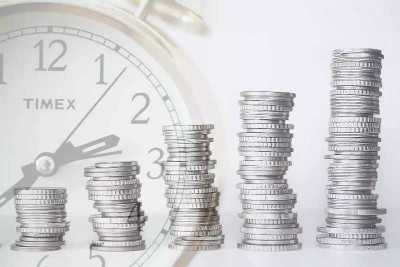# Cost And Price Of National Income (ni)

While calculating national income, the issues related to ‘cost’ and ‘price’ also needs to be decided. Basically, there are two sets of costs and prices; and an economy needs to choose at which of the two costs and two prices it will calculate its National Income.
• NI is equal to the Net National Product (NNP) at Factor Cost.
• GDP is calculated by adding National Private Consumption, Gross Investment, Government spending and Trade Balance (Export-Import). It is the sum of Gross Value added (GVA) at basic price including taxes but excludes product taxes.

COST:
• The income of an economy, i.e., the value of its total produced goods and services may be calculated at either the ‘factor cost’ or the ‘market cost’.
• ‘Factor cost’ is the ‘input cost’ that the producer has to incur in the process of producing something (such as cost of capital, i.e., interest on loans, raw materials, labor, rent, power, etc.). This is also termed as ‘factory price’ or ‘production cost/ price’. This is nothing but ‘price’ of the commodity from the producer’s side.• ‘Market cost’ is derived after adding the indirect taxes to the factor cost of the product, it means the cost at which the goods reach the market, i.e., showrooms (these are the CENVAT/central excise and the CST which are paid by the producers to the central government in India). This is also known as the ‘ex-factory price’.
• Factor Cost = Market Cost – Net Indirect Taxes {Net Indirect Taxes=Indirect Taxes - Subsidies}
• Since 2015, India has switched over to calculating its national income at market cost. This is in line with international best practice. Implementation of GST has eased the process of calculating national income at market price.PRICE:
• Income can be derived at two prices, constant and current.
• The difference in the constant and current prices is only that of the impact of inflation.
• Inflation is considered standstill at a year of the past (this year of the past is also known as the ‘base year’) in the case of the constant price, while in the current price, present day inflation is added.
• Current price is, basically, the maximum retail price (MRP) which is printed on the goods selling in the market.
• India calculates its national income at constant prices—so is the situation among other developing economies, while the developed nations calculate it at the current prices.
• The base year in India has been revised from 2004–05 to 2011–12 (January 2015).